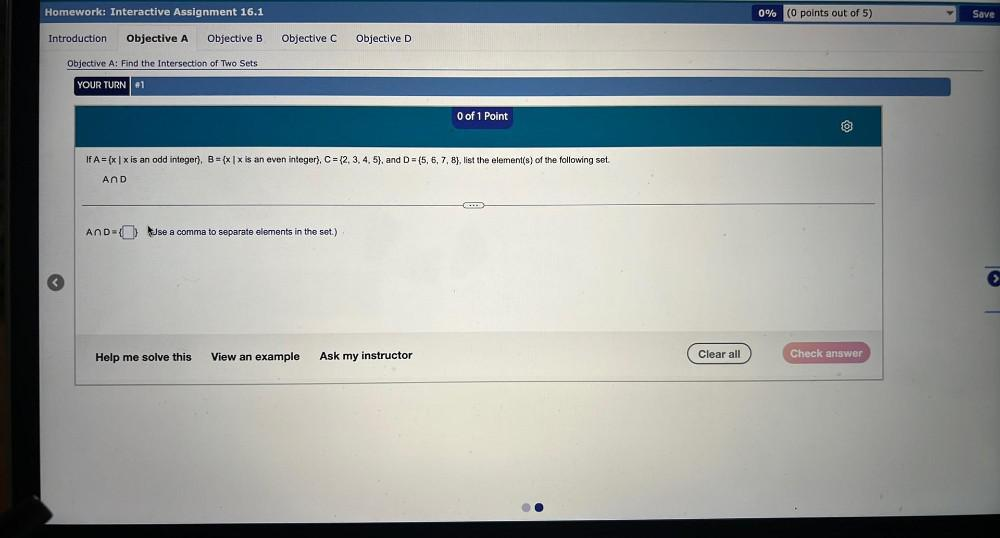Question:

# If A = {x | x is an odd integer), B = {xl x is an even integer}, C = {2, 3, 4, 5), and D = {5, 6, 7, 8), list the element(s) of the following set. a)A∩D=_____If A = {x | x is an odd integer), B = {xl x is an even integer}, C = {2, 3, 4, 5), and D = {5, 6, 7, 8), list the element(s) of the following set. a)A∩D=_____